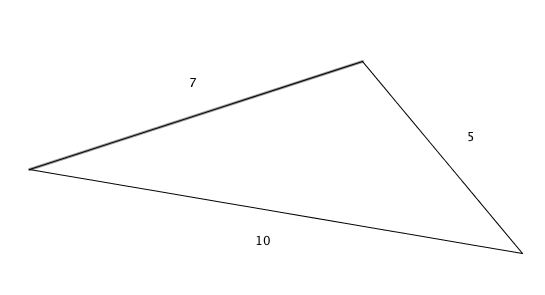Geometry

Perimeter

Khom drew a triangle has side lengths of 5, 7 and 10. What is the perimeter of this triangle?What is the perimeter of a square with side length 3?

What is the perimeter (in inches) of a rectangle which measures 8 inches by 15 inches?

A regular hexagon has a side of length 7. What is its perimeter?

Scalene triangle $ABC$ has side lengths $AB = 10$, $BC = 14$ and $CA = 16$. What is the perimeter of $ABC$?

×